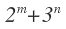# Is the number a square?Find all pairs (m,n) of positive integers for which the above expression is a perfect square.Note by Shashank Rammoorthy
6 years, 8 months ago

This discussion board is a place to discuss our Daily Challenges and the math and science related to those challenges. Explanations are more than just a solution — they should explain the steps and thinking strategies that you used to obtain the solution. Comments should further the discussion of math and science.

When posting on Brilliant:

• Use the emojis to react to an explanation, whether you're congratulating a job well done , or just really confused .
• Ask specific questions about the challenge or the steps in somebody's explanation. Well-posed questions can add a lot to the discussion, but posting "I don't understand!" doesn't help anyone.
• Try to contribute something new to the discussion, whether it is an extension, generalization or other idea related to the challenge.

MarkdownAppears as
*italics* or _italics_ italics
**bold** or __bold__ bold
- bulleted- list
• bulleted
• list
1. numbered2. list
1. numbered
2. list
Note: you must add a full line of space before and after lists for them to show up correctly
paragraph 1paragraph 2

paragraph 1

paragraph 2

[example link](https://brilliant.org)example link
> This is a quote
This is a quote
    # I indented these lines
# 4 spaces, and now they show
# up as a code block.

print "hello world"
# I indented these lines
# 4 spaces, and now they show
# up as a code block.

print "hello world"
MathAppears as
Remember to wrap math in $$ ... $$ or $ ... $ to ensure proper formatting.
2 \times 3 $2 \times 3$
2^{34} $2^{34}$
a_{i-1} $a_{i-1}$
\frac{2}{3} $\frac{2}{3}$
\sqrt{2} $\sqrt{2}$
\sum_{i=1}^3 $\sum_{i=1}^3$
\sin \theta $\sin \theta$
\boxed{123} $\boxed{123}$

Sort by:

Suppose $2^{m}+3^{n}=a^{2}$.

It is easy to show that both $m$ and $n$ are even.Let $m=2r$ and $n=2s$

Hence, $2^{2r}=a^{2}-3^{2s}=(a-3^{s})(a+3^{s})$

Hence, $a-3^{s}=2^{i}$...$(1)$ and $a+3^{s}=2^{2r-i}$...$(2)$

$(2)-(1)$ gives $2.3^{s}=2^{i}(2^{2r-2i}-1)$, which implies $i=1$.

Thus, $a-3^{s}=2$ and $a+3^{s}=2^{2r-1}$.Hence, $3^{s}=2^{2r-2}-1$...$(3)$

Suppose, $s>1$.Then $r≥3$.But then the equation $(3)$ is impossible since when divided by $8$, the left hand side $3^{s}$ leaves a remainder $1$ or $3$ while the right hand side would leave the remainder $7$.Thus $s=1$ is the only possibility.When $s=1$,i.e, $n=2$,we have the solution $2^{4}+3^{2}=25$.Thus $(m,n)=(4,2)$ is the only solution.

- 6 years, 8 months ago

Thank you for this solution! Been thinking about this all day. Never got the chance to sit down with a pen and paper, unfortunately...

- 6 years, 8 months ago

How to show that both $m$ and $n$ are even?

- 6 years, 8 months ago

$a^{2}≡0 or 1(mod 3)$ and $2^{m}+3^{n}≡2^{m}(mod 3)$.But $2^{m}$ is not congruent $0$ modulo $3$.

So,$2^{m}≡1(mod 3)$ which implies $m$ is even.Hence, $3^{n}≡a^{2}≡0 or 1 (mod 4)$.But $4$ does not divides $3^{n}$.So,$3^{n}≡1(mod4)$ which implies $n$ is even.

- 6 years, 8 months ago

How about $(3,0)$ and $(0,1)$ ?

- 6 years, 8 months ago

m, n are positive integers. 0 is not positive.

- 6 years, 8 months ago

Could you please explain your solution from line 5 onwards? How is it 2.3^s? Thanks.

- 6 years, 8 months ago

I just subtracted eqn $1$ from eqn $2$ and got $2\times3^{s}=2^{2r-i}-2^{i}=2^{i}(2^{2r-2i}-1)$.

Hence, $3^{s}=2^{i-1}(2^{2r-2i}-1)$.If $i>1$,then $2$ divides $3^{s}$, which is impossible.So $i=1$.

So,$3^{s}=2^{1-1}(2^{2r-2.1}-1)=2^{2r-2}-1$, which is eqn $3$.Then the solution is very clear.

- 6 years, 8 months ago

Thanks.

- 6 years, 8 months ago

(4,2),(3,0),(0,1),

- 6 years, 8 months ago